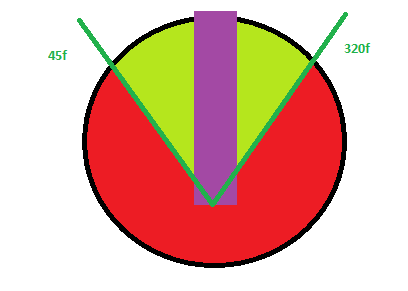# Limiting top down rotation amount - 2d

Hi Guys, I am stumped with a simple piece of logic which I hope someone can help me with.

I have a top down driving game where I want a wheel only to turn between an eulerAngler of 40f - 320f

I have drawn a simple diagram to explain below:I’ve tried many things but this is as far as I got…

``````public float speed = 5.0f;

void FixedUpdate () {
float angle = transform.rotation.eulerAngles.z;
float turnForce = Input.GetAxis("Horizontal") * speed;

if ( angle <= 40f && turnForce < 0)
{
transform.Rotate(0.0f, 0.0f, turnForce);
}

if (angle >= 320f && turnForce > 0)
{
transform.Rotate(0.0f, 0.0f, turnForce);
}
``````

Can anyone help?

Thanks

Ryan

bump?has no one have any idea?